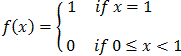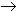#Interactive Real Analysis

Next | Previous | Glossary | Map

## 8.1. Pointwise Convergence

### Example 8.1.8 (a): Pointwise Convergence does not preserve Continuity

Find a pointwise convergent sequence of functions, each of which is continuous, but whose limit function is not continuous.

 Let fn(x) = xn for 0[0, 1] and defineThen: each fn(x) is continuous (even differentiable) fn(x)f pointwise the limit function f is not continuous at x=1 Thus, pointwise convergence does not preserve continuity.
Next | Previous | Glossary | Map